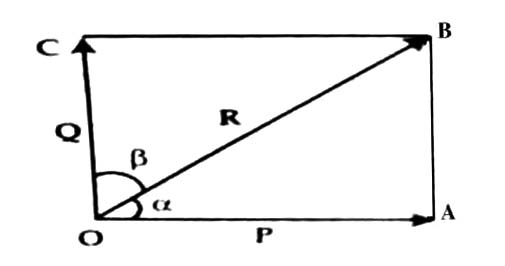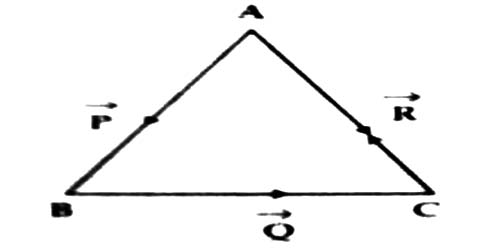# Vectors Addition and Subtraction in terms of Perpendicular Components

Vectors addition and subtraction in terms of components

A vector quantity can be resolved by parallelogram law into two or more vectors in different directions. The process of resolving a vector into two or more vectors is called resolution of a vector or vector resolution. Each resolving vector is called component of the original vector.

Resolution into perpendicular components:

Let R be the vector along OB, so that OB = R. Now taking OB as diagonal let us draw the rectangle OABC [Fig. 1]. Here the components P and Q are perpendicular to one another, i.e.,

α + β = 900

then, Sin (α + β) = Sin 900 = 1 and;

Sin β = Sin (900 – α) = Cos αAccording to law at trainee at trigonometry we get from the triangle OAB.

P/Cos θ = Q/Sin θ = R/Sin 900

so, P = R Cos θ and Q = R sin α … …. ….. (1)

The two components P and Q are called the perpendicular components of the resultant R. P is called the horizontal component and Q is called tangential component.

Vector forms of the two components are –

P = R Cos α î + R Sin α î